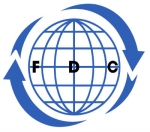Search all upcoming seminars, conferences, short management courses and training in Nigeria and around the World# Quantitative Data Management and Analysis with R Course

## By: Foscore Development Center

Kenya

02 - 06 Mar, 2020  5 days

### USD 2,000

Venue: Foscore Development Center, Nairobi, Kenya

This course is designed for participants who plan to use R for the management, coding, analysis and visualization of qualitative data. The course’s content is spread over seven modules and includes: Basics of Applied Statistical Modelling, Essentials of the R Programming, Statistical Tools, Probability Distributions, Statistical Inference, Relationship between Two Different Quantitative Variables and Multivariate Analysis. The course is entirely hands-on and uses sample data to learn R basics and advanced features.

Who Should Attend?

Statistician, analyst, or a budding data scientist and beginners who want to learn how to analyze data with R,

Course Objective:

• Analyze t data by applying appropriate statistical techniques
• Interpret the  statistical analysis
• Identify statistical techniques a best suited to data and questions
• Strong foundation in fundamental statistical concepts
• Implement different statistical analysis in R and interpret the results
• Build intuitive data visualizations
• Carry out formalized hypothesis testing
• Implement linear modelling techniques such multiple regressions and GLMs
• Implement advanced regression analysis and multivariate analysis

Course Content

Module One: Basics of Applied Statistical Modelling

• Introduction to the Instructor and Course
• Data and Code Used in the Course
• Statistics in the Real World
• Designing Studies and Collecting Good Quality Data
• Different Types of Data

Module Two: Essentials of the R Programming

• Rationale for this section
• Introduction to the R Statistical Software and R Studio
• Different Data Structures in R
• Reading in Data from Different Sources
• Indexing and Subletting of Data
• Data Cleaning: Removing Missing Values
• Exploratory Data Analysis in R

Module Three: Statistical Tools

• Quantitative Data
• Measures of Center
• Measures of Variation
• Charting and Graphing Continuous Data
• Charting and Graphing Discrete Data
• Deriving Insights from Qualitative/Nominal Data

Module Four: Probability Distributions

• Data Distribution: Normal Distribution
• Checking For Normal Distribution
• Standard Normal Distribution and Z-scores
• Confidence Interval-Theory
• Confidence Interval-Computation in R

Module Five: Statistical Inference

• Hypothesis Testing
• T-tests: Application in R
• Non-Parametric Alternatives to T-Tests
• One-way ANOVA
• Non-parametric version of One-way ANOVA
• Two-way ANOVA
• Power Test for Detecting Effect

Module Six: Relationship between Two Different Quantitative Variables

• Explore the Relationship Between Two Quantitative Variables
• Correlation
• Linear Regression-Theory
• Linear Regression-Implementation in R
• Conditions of Linear Regression
• Multi-collinearity
• Linear Regression and ANOVA
• Linear Regression With Categorical Variables and Interaction Terms
• Analysis of Covariance (ANCOVA)
• Selecting the Most Suitable Regression Model
• Violation of Linear Regression Conditions: Transform Variables
• Other Regression Techniques When Conditions of OLS Are Not Met
• Regression: Standardized Major Axis (SMA) Regression
• Polynomial and Non-linear regression
• Linear Mixed Effect Models
• Generalized Regression Model (GLM)
• Logistic Regression in R
• Poisson Regression in R
• Goodness of fit testing

Module Seven: Multivariate Analysis

• Introduction Multivariate Analysis
• Cluster Analysis/Unsupervised Learning
• Principal Component Analysis (PCA)
• Linear Discriminant Analysis (LDA)
• Correspondence Analysis
• Similarity and Dissimilarity Across Sites
• Non-metric multi-dimensional scaling (NMDS)
• Multivariate Analysis of Variance (MANOVA)

Note

• All our courses can be Tailor-made to participants needs
• Course duration is flexible and the contents can be modified to fit any number of days.
• Presentations are well guided, practical exercise, web based tutorials and group work. Our facilitators are expert with more than 10years of experience.
• One year free Consultation and Coaching provided after the course.
 Foscore Development Center, Nairobi, Kenya Mar 02 - 06 Mar, 2020
 USD 2,000.00
(Convert Currency)

Jackson Munene +254 712260031

A discount of 10% to 50% for a group of more than two
Foscore Development Center